# Analyzing selling price of used cars using Python

Now-a-days, with the technological advancement, Techniques like Machine Learning, etc are being used on a large scale in many organisations. These models usually work with a set of predefined data-points available in the form of datasets. These datasets contain the past/previous information on a specific domain. Organising these datapoints before it is fed to the model is very important. This is where we use Data Analysis. If the data fed to the machine learning model is not well organised, it gives out false or undesired output. This can cause major losses to the organisation. Hence making use of proper data analysis is very important.

The data that we are going to use in this example is about cars. Specifically containing various information datapoints about the used cars, like their price, color, etc. Here we need to understand that simply collecting data isn’t enough. Raw data isn’t useful. Here data analysis plays a vital role in unlocking the information that we require and to gain new insights into this raw data.

Consider this scenario, our friend, Otis, wants to sell his car. But he doesn’t know how much should he sell his car for! He wants to maximize the profit but he also wants it to be sold for a reasonable price for someone who would want to own it. So here, us, being a data scientist, we can help our friend Otis.

Let’s think like data scientists and clearly define some of his problems: For example, is there data on the prices of other cars and their characteristics? What features of cars affect their prices? Colour? Brand? Does horsepower also affect the selling price, or perhaps, something else?

As a data analyst or data scientist, these are some of the questions we can start thinking about. To answer these questions, we’re going to need some data. But this data is in raw form. Hence we need to analyze it first. The data is available in the form of `.csv/.data` format with us

To download the file used in this example click here. The file provided is in the .data format. Follow the below process for converting a .data file to .csv file.

Process to convert .data file to .csv:

1. open MS Excel
2. Go to DATA
3. Select From text
4. Check box tick on comas(only)

Modules needed:

• pandas: Pandas is an opensource library that allows you to perform data manipulation in Python. Pandas provide an easy way to create, manipulate and wrangle the data.
• numpy: Numpy is the fundamental package for scientific computing with Python. `numpy `can be used as an efficient multi-dimensional container of generic data.
• matplotlib: Matplotlib is a Python 2D plotting library which produces publication quality figures in a variety of formats.
• seaborn: Seaborn is a Python data-visualization library that is based on matplotlib. Seaborn provides a high-level interface for drawing attractive and informative statistical graphics.
• scipy: Scipy is a Python-based ecosystem of open-source software for mathematics, science, and engineering.

Steps for installing these packages:

• If you are using anaconda- jupyter/ syder or any other third party softwares to write your python code, make sure to set the path to the “scripts folder” of that software in command prompt of your pc.
• Then type – pip install package-name
Example:

```pip install numpy
```
• Then after the installation is done. (Make sure you are connected to the internet!!) Open your IDE, then import those packages. To import, type – import package name
Example:

```import numpy
```

Steps that are used in the following code (Short description):

• Import the packages
• Set the path to the data file(.csv file)
• Find if there are any null data or NaN data in our file. If any, remove them
• Perform various data cleaning and data visualisation operations on your data. These steps are illustrated beside each line of code in the form of comments for better understanding, as it would be better to see the code side by side than explaining it entirely here, would be meaningless.
• Obtain the result!

Lets start analyzing the data.

Step 1: Import the modules needed.

 `# importing section ` `import` `pandas as pd  ` `import` `numpy as np ` `import` `matplotlib.pyplot as plt ` `import` `seaborn as sns ` `import` `scipy as sp `

Step 2: Let’s check the first five entries of dataset.

 `# using the Csv file ` `df ``=` `pd.read_csv(``'output.csv'``)  ` ` `  `# Checking the first 5 entries of dataset ` `df.head() `

Output: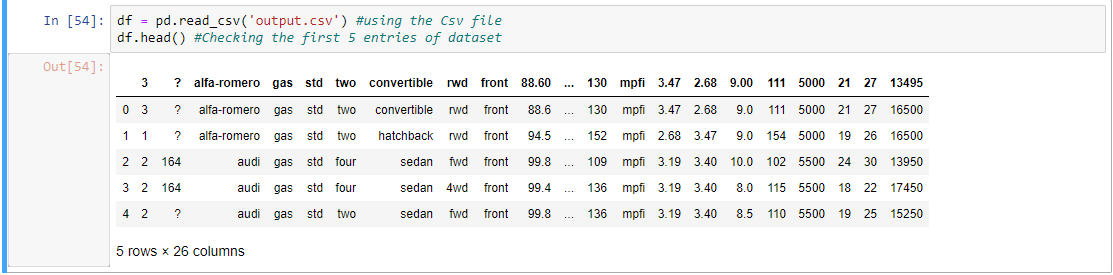Step 3: Defining headers for our dataset.

 `headers ``=` `[``"symboling"``, ``"normalized-losses"``, ``"make"``,  ` `           ``"fuel-type"``, ``"aspiration"``,``"num-of-doors"``, ` `           ``"body-style"``,``"drive-wheels"``, ``"engine-location"``, ` `           ``"wheel-base"``,``"length"``, ``"width"``,``"height"``, ``"curb-weight"``, ` `           ``"engine-type"``,``"num-of-cylinders"``, ``"engine-size"``,  ` `           ``"fuel-system"``,``"bore"``,``"stroke"``, ``"compression-ratio"``, ` `           ``"horsepower"``, ``"peak-rpm"``,``"city-mpg"``,``"highway-mpg"``,``"price"``] ` ` `  `df.columns``=``headers ` `df.head() `

Output: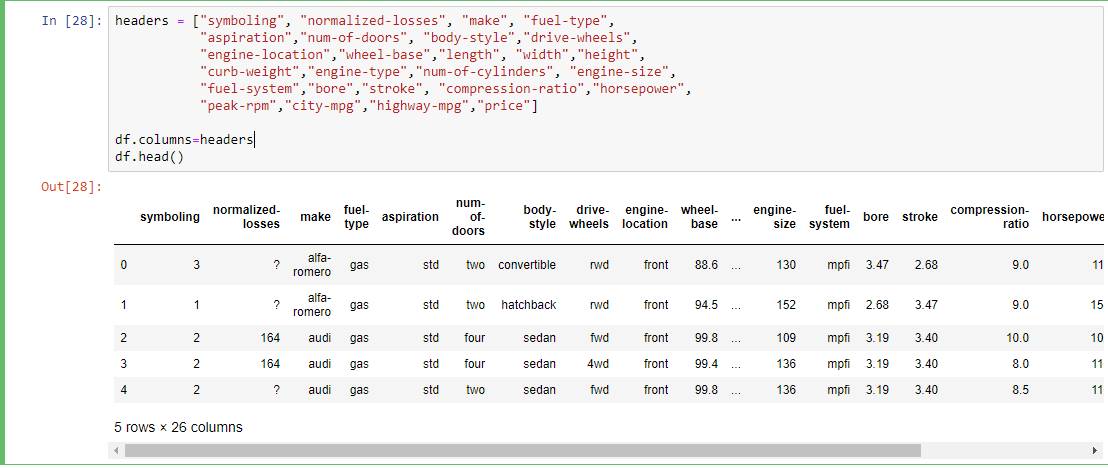Step 4: Finding the missing value if any.

 `data ``=` `df ` ` `  `# Finding the missing values ` `data.isna().``any``() ` ` `  `# Finding if missing values  ` `data.isnull().``any``()  `

Output: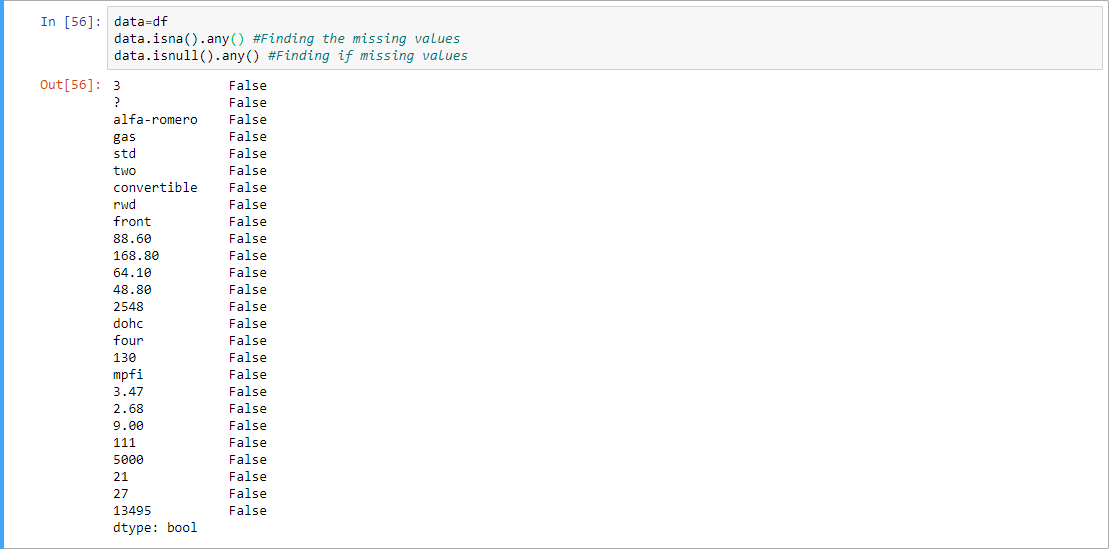Step 4: Converting mpg to L/100km and checking the data type of each column.

 `# converting mpg to L / 100km ` `data[``'city-mpg'``] ``=` `235` `/` `df[``'city-mpg'``] ` `data.rename(columns ``=` `{``'city_mpg'``: ``"city-L / 100km"``}, inplace ``=` `True``) ` ` `  `print``(data.columns) ` ` `  `# checking the data type of each column ` `data.dtypes  `

Output: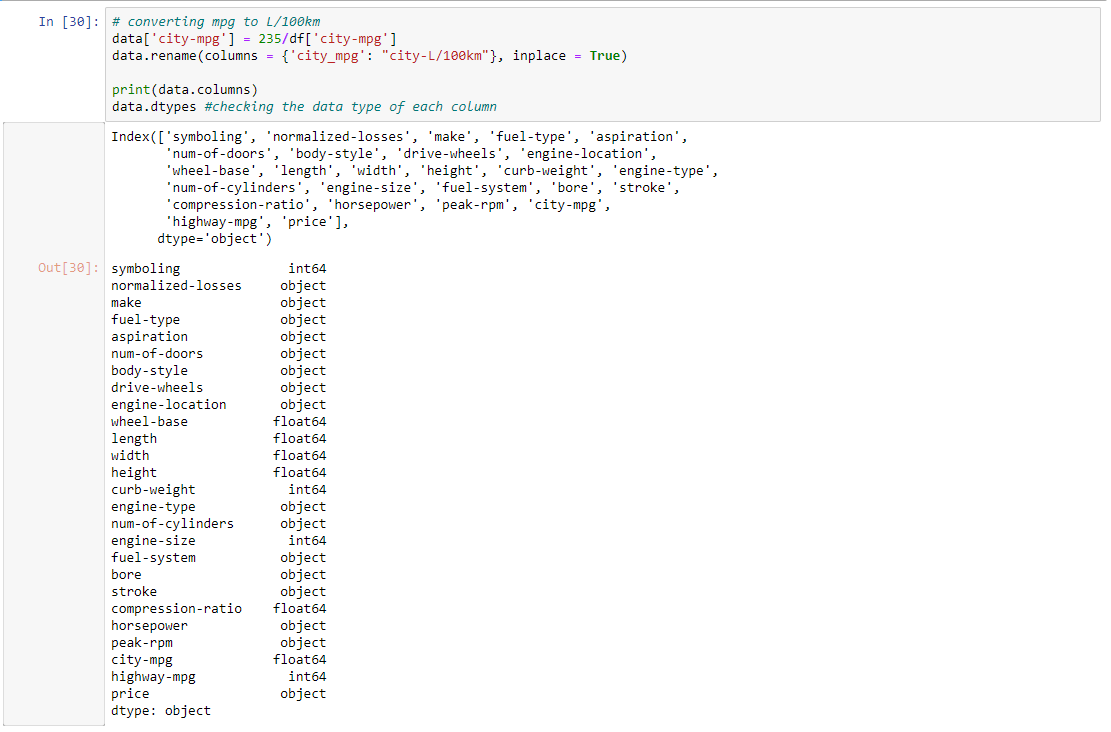Step 5: Here, price is of object type(string), it should be int or float, so we need to change it

 `data.price.unique() ` ` `  `# Here it contains '?', so we Drop it ` `data ``=` `data[data.price !``=` `'?'``] ` ` `  `# checking it again ` `data.dtypes `

Output: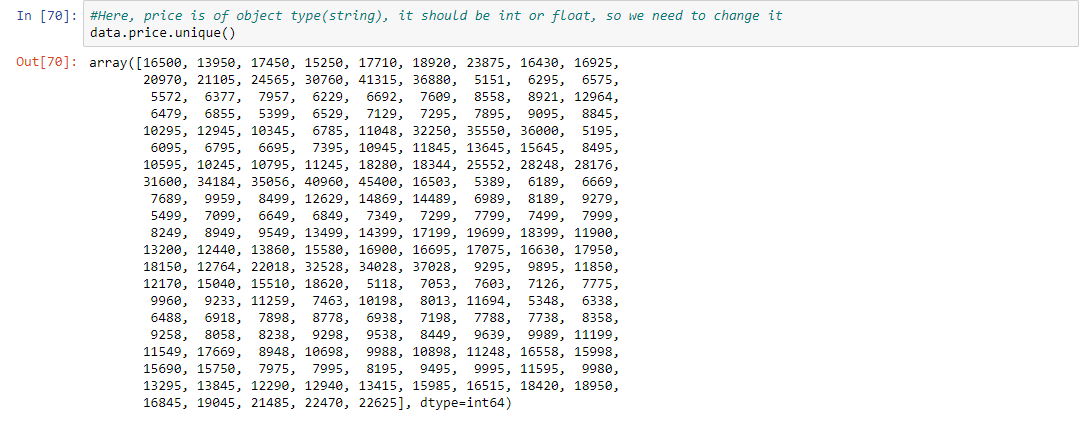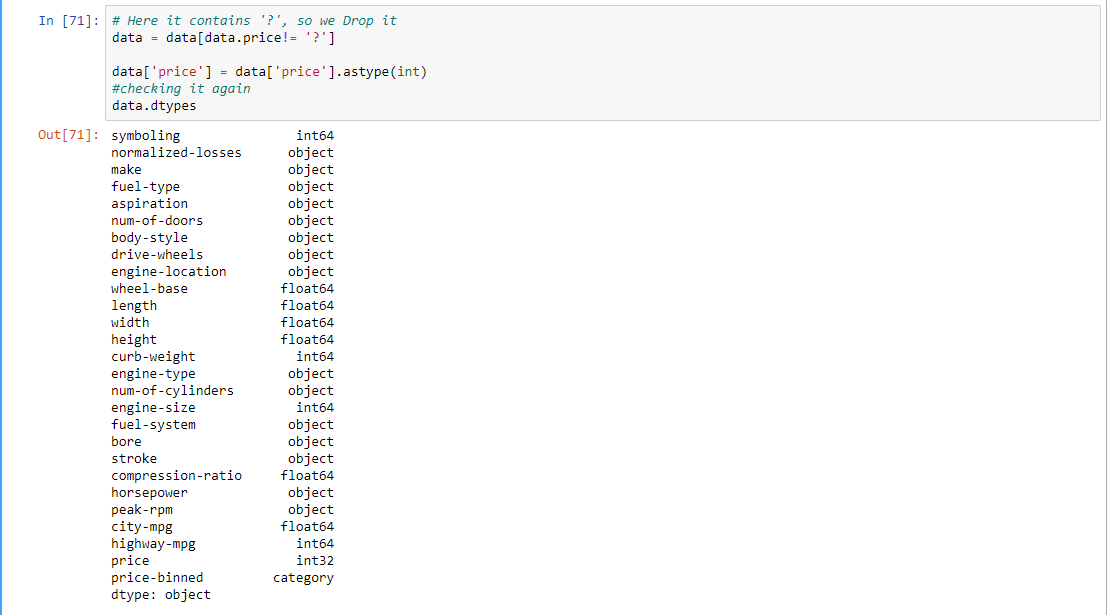Step 6: Normalizing values by using simple feature scaling method examples(do for the rest) and binning- grouping values

 `data[``'length'``] ``=` `data[``'length'``]``/``data[``'length'``].``max``() ` `data[``'width'``] ``=` `data[``'width'``]``/``data[``'width'``].``max``() ` `data[``'height'``] ``=` `data[``'height'``]``/``data[``'height'``].``max``() ` ` `  `# binning- grouping values ` `bins ``=` `np.linspace(``min``(data[``'price'``]), ``max``(data[``'price'``]), ``4``)  ` `group_names ``=` `[``'Low'``, ``'Medium'``, ``'High'``] ` `data[``'price-binned'``] ``=` `pd.cut(data[``'price'``], bins,  ` `                              ``labels ``=` `group_names,  ` `                              ``include_lowest ``=` `True``) ` ` `  `print``(data[``'price-binned'``]) ` `plt.hist(data[``'price-binned'``]) ` `plt.show() `

Output: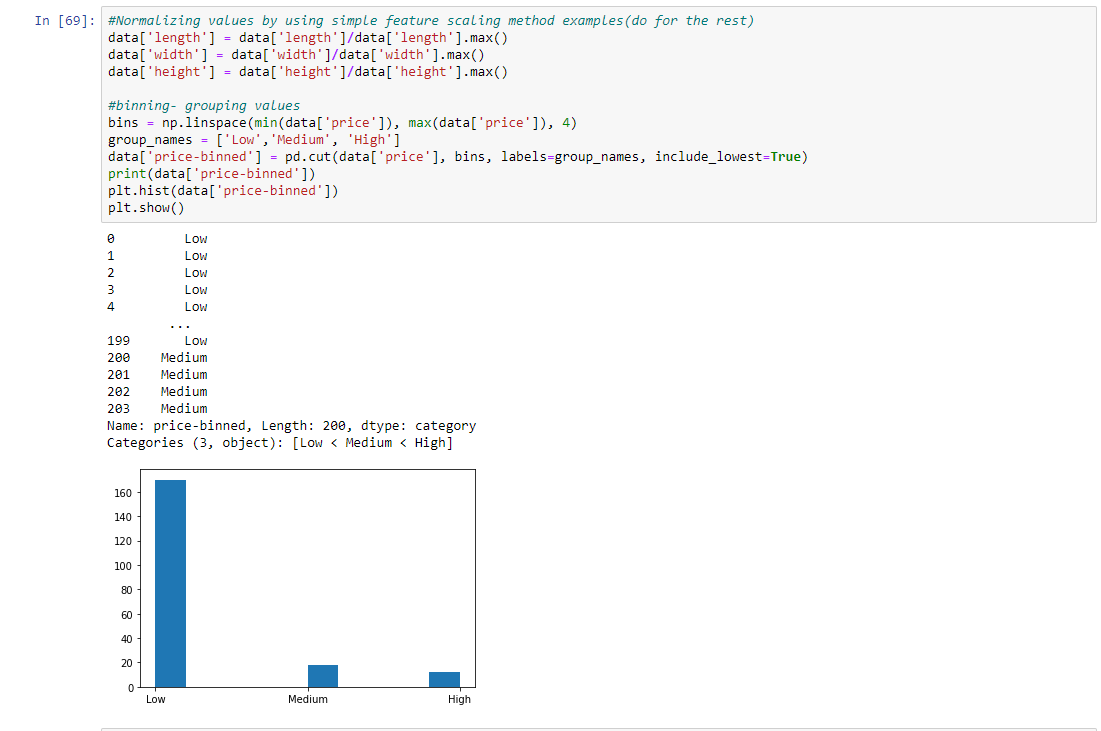Step 7: Doing descriptive analysis of data categorical to numerical values.

 `# categorical to numerical variables ` `pd.get_dummies(data[``'fuel-type'``]).head() ` ` `  `# descriptive analysis ` `# NaN are skipped ` `data.describe() `

Output: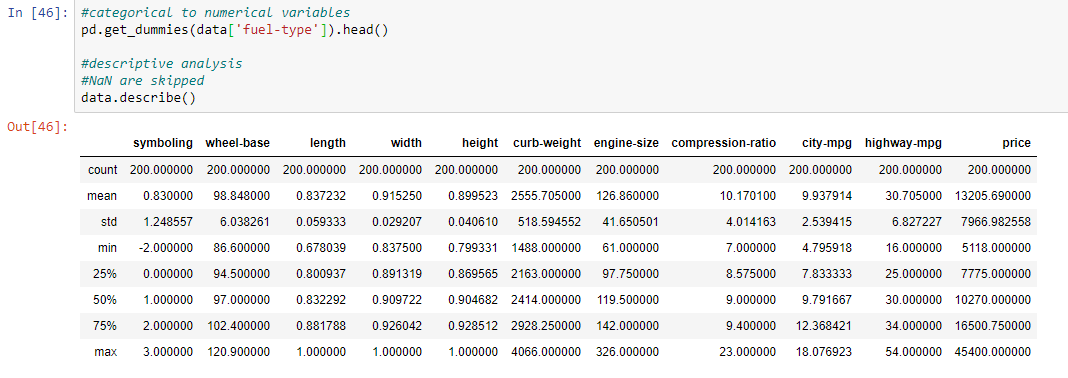Step 8: Plotting the data according to the price based on engine size.

 `# examples of box plot ` `plt.boxplot(data[``'price'``]) ` ` `  `# by using seaborn ` `sns.boxplot(x ``=``'drive-wheels'``, y ``=``'price'``, data ``=` `data) ` ` `  `# Predicting price based on engine size ` `# Known on x and predictable on y ` `plt.scatter(data[``'engine-size'``], data[``'price'``]) ` `plt.title(``'Scatterplot of Enginesize vs Price'``) ` `plt.xlabel(``'Engine size'``) ` `plt.ylabel(``'Price'``) ` `plt.grid() ` `plt.show() `

Output: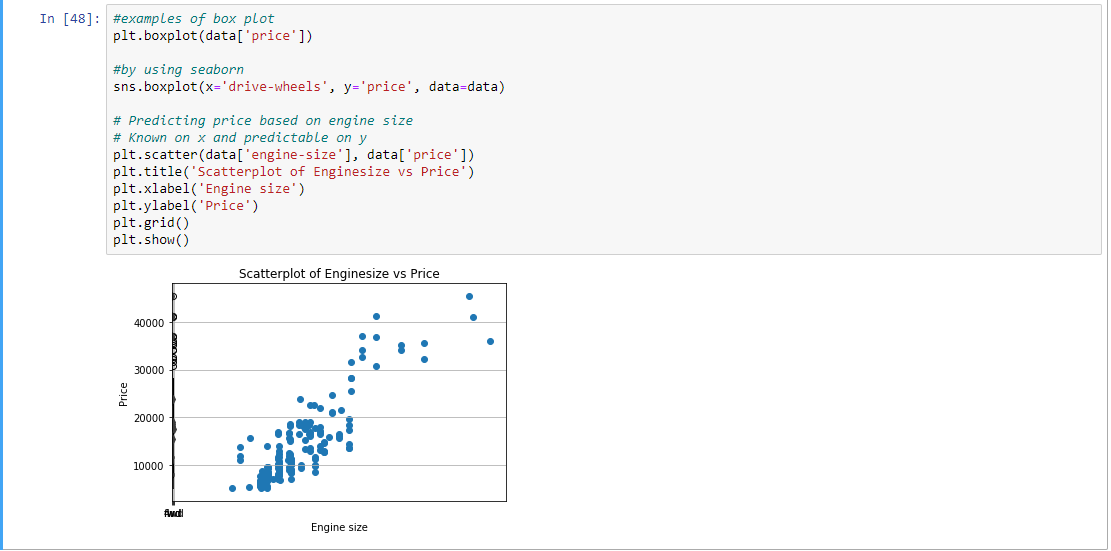Step 9: Grouping the data according to wheel, body-style and price.

 `# Grouping Data ` `test ``=` `data[[``'drive-wheels'``, ``'body-style'``, ``'price'``]] ` `data_grp ``=` `test.groupby([``'drive-wheels'``, ``'body-style'``],  ` `                         ``as_index ``=` `False``).mean() ` ` `  `data_grp `

Output: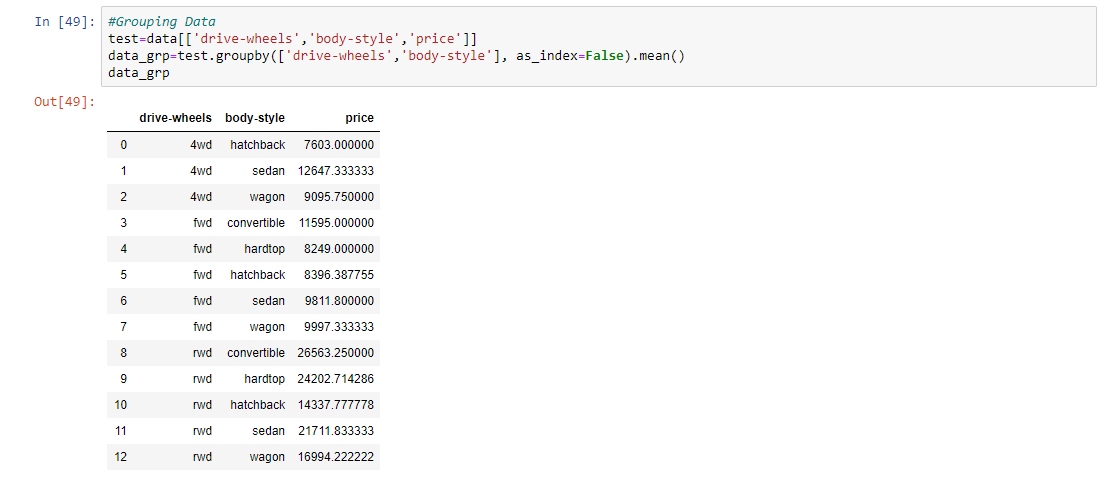Step 10: Using the pivot method and plotting the heatmap according to the data obtained by pivot method

 `# pivot method ` `data_pivot ``=` `data_grp.pivot(index ``=` `'drive-wheels'``, ` `                            ``columns ``=` `'body-style'``) ` `data_pivot ` ` `  `# heatmap for visualizing data ` `plt.pcolor(data_pivot, cmap ``=``'RdBu'``) ` `plt.colorbar() ` `plt.show() `

Output: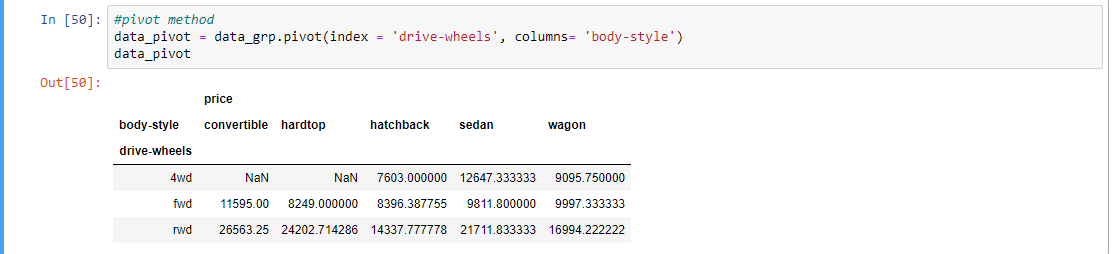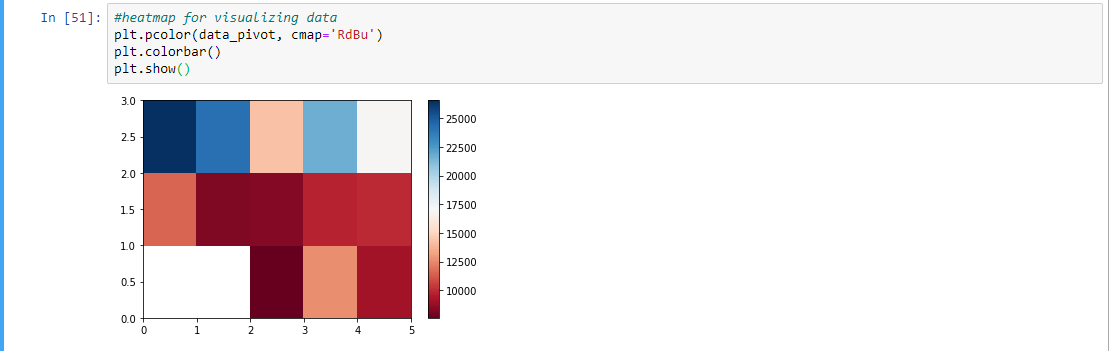Step 11: Obtaining the final result and showing it in the form of a graph. As the slope is increasing in a positive direction, it is a positive linear relationship.

 `# Analysis of Variance- ANOVA ` `# returns f-test and p-value ` `# f-test = variance between sample group means divided by  ` `# variation within sample group ` `# p-value = confidence degree ` `data_annova ``=` `data[[``'make'``, ``'price'``]] ` `grouped_annova ``=` `data_annova.groupby([``'make'``]) ` `annova_results_l ``=` `sp.stats.f_oneway( ` `                             ``grouped_annova.get_group(``'honda'``)[``'price'``], ` `                             ``grouped_annova.get_group(``'subaru'``)[``'price'``] ` `                                    ``) ` `print``(annova_results_l) ` ` `  `# strong corealtion between a categorical variable ` `# if annova test gives large f-test and small p-value ` ` `  `# Correlation- measures dependency, not causation ` `sns.regplot(x ``=``'engine-size'``, y ``=``'price'``, data ``=` `data) ` `plt.ylim(``0``, ) `

Output: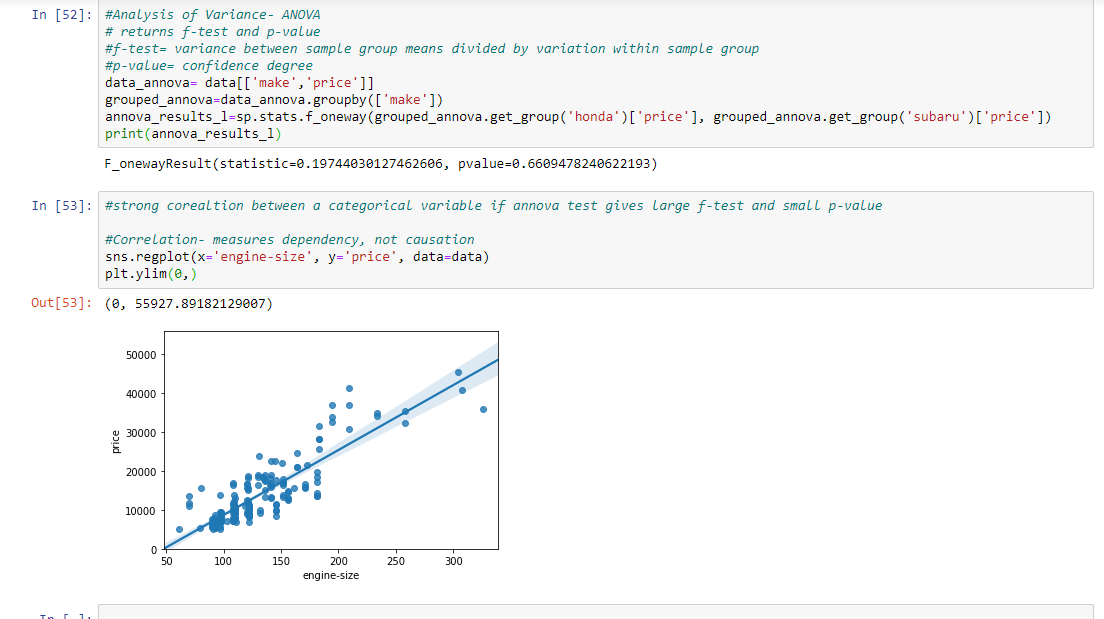My Personal Notes arrow_drop_upCheck out this Author's contributed articles.

If you like GeeksforGeeks and would like to contribute, you can also write an article using contribute.geeksforgeeks.org or mail your article to contribute@geeksforgeeks.org. See your article appearing on the GeeksforGeeks main page and help other Geeks.

Please Improve this article if you find anything incorrect by clicking on the "Improve Article" button below.

Article Tags :

10

Please write to us at contribute@geeksforgeeks.org to report any issue with the above content.